# Test: Mixtures- 1

## 15 Questions MCQ Test Quantitative Reasoning for GMAT | Test: Mixtures- 1

Description
Attempt Test: Mixtures- 1 | 15 questions in 40 minutes | Mock test for GMAT preparation | Free important questions MCQ to study Quantitative Reasoning for GMAT for GMAT Exam | Download free PDF with solutions
QUESTION: 1

Solution:
QUESTION: 2

Solution:
QUESTION: 3

### A 30% alcohol solution (only alcohol & water) is poured into a container having a 60% alcohol solution (only alcohol & water). If the resultant mixture has 50% alcohol, then what is the ratio in which the 30% solution is mixed with the 60% Alcohol solution?

Solution:
QUESTION: 4

A solution X is 80% A and 20% B. Another solution Y is 60% B and 40% C. If 6 litres of Solution X is mixed with 2 litres of Solution Y, then what is the concentration of compound B in the final solution?

Solution:
QUESTION: 5

A juicy fruit X contains 70% water and remaining is pulp. If the fruit X is dried to remove the entire water and now weighs 180 grams, what was the original weight of the fruit?

Solution:
QUESTION: 6

How many liters of a 60% alcohol solution must be added to 50 liters of a 40% alcohol solution to result in 50% alcohol solution?

Solution:

Step 1: Question statement and Inferences

Let x liters of the 60% solution be added.

So, amount of Alcohol in this solution = 60% of x = 0.6x L

Now, let us consider the 40% solution.

The total volume of this solution is 50 L

So, amount of Alcohol in this solution = 40% of 50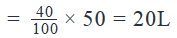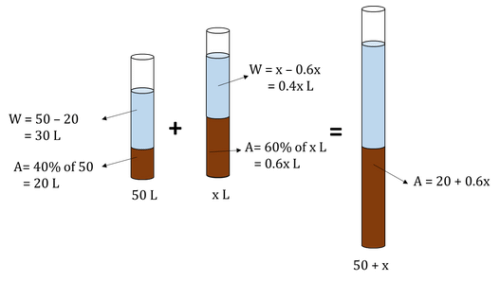Step 2: Finding required values

When x L of 60% solution are mixed with 50 L of the 40% solution, the total volume of the resultant solution will be (50+x) L

By the Law of Conservation of Mass,

Amount of alcohol in the input solutions = Amount of alcohol in the final solution

=> Amount of alcohol in the final solution = 20 + 0.6x

So, concentration of Alcohol in the final solution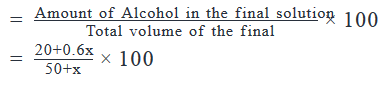Step 3: Calculating the final answer

It is given that the concentration of alcohol in the final solution is 50%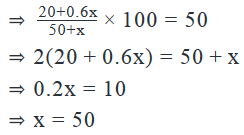QUESTION: 7

How much water should be added (in liters) to 2 liters of milk having 3% fat to reduce the concentration of fat to 1% by volume?

Solution:

Step 1: Question statement and Inferences

The question is how much water you need to add to dilute the milk. The most important thing you should remember is that the final mixture volume will be the starting 2 liters plus the liters that you add. We will draw a diagram of the mixture and the changes done to it, to help us set the equations.

Step 2: Finding required values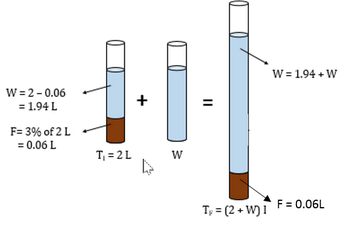Initially, in the mixture,

Amount of fat = 3% of 2L = 0.06 L

Let the amount of water added = W liters

So, the total volume of the solution is 2 + W liters.

But, the volume of fat is still 0.06 L

So, the new concentration of fat in the solution, C’ will be equal to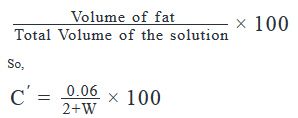It is given that C’ = 1 %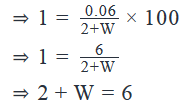Step 3: Calculating the final answer

From the above equation, it’s easy to see that W = 4 liters

QUESTION: 8

A Lentil mixture is prepared using 2 different types of lentils: yellow and red. The ratio, in grams, of yellow lentils to red lentils is 3:2. How many grams of yellow lentils are in the mixture?

(1) The ratio of the densities (mass per unit volume) of yellow lentil to red lentil is 2:3.

(2) If 50 grams of yellow lentils are removed from the mixture, the ratio of yellow to red in the resulting mixture is 5:4

Solution:

Steps 1 & 2: Understand Question and Draw Inferences

The question is asking for the grams of yellow lentils in the mixture. You already have the ratio of grams of lentils, but you need the total number of grams.

Let the weight of the yellow lentils be 3x grams, where x is an unknown number

Thus, weight of the red lentils = 2x grams

And, weight of the mixture = 3x + 2x = 5x grams

Thus, in order to find the weight of the yellow lentils, we need to find the value of x.

Step 3: Analyze Statement 1

1. The ratio of the densities of yellow lentil to red lentil is 2:3.

This tells you that the red lentils are more dense than the yellow lentils, so they take up less space. If you had the total volume of lentils, this may help you, but what you need is the total weight.

Statement 1 is not sufficient.

Step 4: Analyze Statement 2

2. If 50 grams of yellow lentils are removed from the mixture, the ratio of yellow to red in the resulting mixture is 5:4

When 50 grams of yellow lentils are removed,

Weight of yellow lentils left, Y’ = (3x – 50) grams

Weight of red lentils is still 2x grams.

We are given that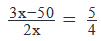This is a linear equation with one unknown. By solving this equation, we will be able to determine a unique value of x.

Thus,

Statement (2) is sufficient.

Step 5: Analyze Both Statements Together (if needed)

You get a unique answer in step 4, so this step is not required

QUESTION: 9

A solution of salt and water has a volume of 1 Gallon. The solution is heated on the flame and consequently the volume of the solution decreased because some water evaporated. What is the percentage of salt by volume in the resultant solution?

(1) The initial solution contained 10% salt by volume.

(2) The volume of the final solution is 3/4 Gallon.

Solution:

Steps 1 & 2: Understand Question and Draw Inferences

In the initial solution, let the amount of Salt be x Gallon

So, the amount of Water will be (1-x) Gallon

As a result of heating this solution on the flame, some water evaporates.

Let the volume of evaporated water be y Gallon

So, the amount of water left = (1 – x – y) Gallons

The amount of salt is still x Gallons.

Total volume of the solution left = (1-y ) Gallons

So, the percentage of salt by volume in the final solution =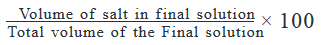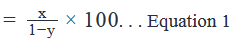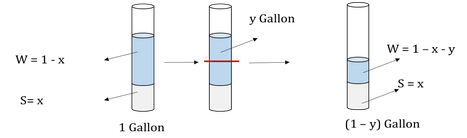Thus, in order to find the percentage of salt by volume in the final solution, we need to know the value of x and y.

Step 3: Analyze Statement 1

As per Statement (1),

The initial solution contained 10% salt by volume.

=> x = 10% of 1 Gallon

From this equation, we will be able to find the value of x. But we get no clue about the value of y.

Therefore,

Statement (1) is not sufficient.

Step 4: Analyze Statement 2

As per Statement (2),

The volume of the final solution is ¾ Gallon.

=> 1 -y = 3/4

=> y = 1/4 Gallon

Thus, Statement (2) gives us the value of y. But we have no clue about the value of x.

Therefore,

Statement (2) is not sufficient.

Step 5: Analyze Both Statements Together (if needed)

From Statement (1),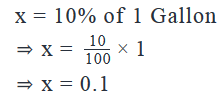From Statement (2),

y = 1/4

Now that we have the values of both x and y, from Equation 1, we will be able to find the percentage of salt by volume in the final solution

Thus, both statements taken together are sufficient to arrive at a unique solution.

QUESTION: 10

A smoothie with 2% strawberry syrup and 5% mango syrup is made in milk. How much more strawberry syrup (in litres) must be added to 12 litres of smoothie so that the smoothie contains 4% strawberry syrup by volume?

Solution:

Step 1: Question statement and Inferences

In order to understand the given information clearly, it will be a good idea to represent the given smoothie and the changes made to it visually.

Step 2: Finding required values

Strawberry syrup accounts for 2% by volume of the 12 L smoothie.

This means, that the amount of strawberry syrup in the smoothie = 2% of 12 = 0.24 L

Let y litres of strawberry syrup be added to the smoothie.

So, the total volume of the smoothie is now (12+y) L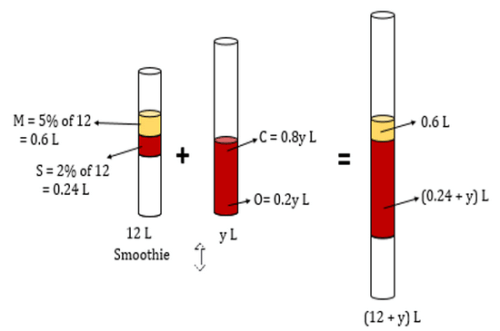Amount of strawberry syrup in the input solutions = Amount of strawberry syrup in the final solution

So, Amount of strawberry syrup in the final solution = 0.24 + y

So, percentage of strawberry syrup in the final smoothie =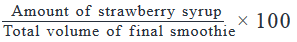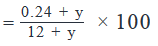Step 3: Calculating the final answer

We are given that the percentage of strawberry syrup in the final smoothie is 4%

So,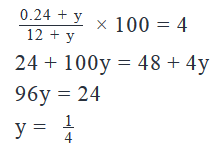QUESTION: 11

Juice A contains 60% orange juice, 20% apple juice and 20% carrot juice.  Juice B contains 20% orange juice and 80% carrot juice.  If a juice concoction is made such that it contains 50% carrot juice, how much juice A is added as a percentage of the juice concoction?

Solution:

Step 1: Question statement and Inferences

Let x liters of Juice A and y liters of Juice B be added to make the juice concoction.

So, the total volume of the juice concoction will be (x + y) L.

We need to find how much of juice A is added as a percentage of the juice concoction such that juice concoction contains 50% carrot juice.

That is, we need to find the value of the expression: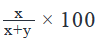So, we will first need to find the values of x and y. Even if we don’t find the exact values of x and y, but are able to find the ratio of x and y, we will still be able to solve this expression.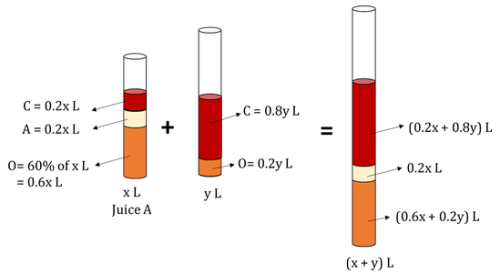Step 2: Finding required values

The amount of carrot juice in Juice A = 20% of x = 0.2x L

The amount of carrot juice in Juice B = 80% of y = 0.8y L

By the Law of Conservation of Mass:

The amount of Carrot Juice in the input solutions = the amount of Carrot Juice in the final solution

So, the amount of carrot juice in the final solution = (0.2x + 0.8y) L

So, the concentration of carrot juice in the final solution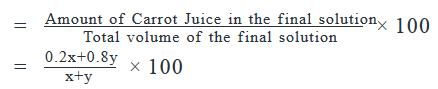Step 3: Calculating the final answer

It is given that the concentration of carrot juice in the final solution is 50%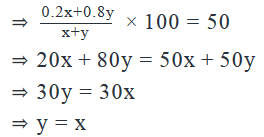therefore,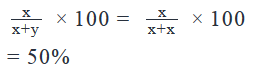For the juice concoction to have 50% carrot juice, Juice A needs to form 50% of the Juice Concoction.

QUESTION: 12

A box initially contains 300 balls of which 40% are red and the rest are green. 50% more red balls are then added to the box. By what percent should the number of red balls be increased further to decrease the percent of green balls to 40%?

Solution:

Step 1: Question statement and Inferences

This is a mixtures problem and is best understood and solved by representing the given information visually.

Step 2: Finding required values

The box contains 300 balls in total.

Red balls account for 40% of this 300.

So, number of Red balls = 40/100 * 300 = 120

The number of Green Balls = 300 – 120 = 180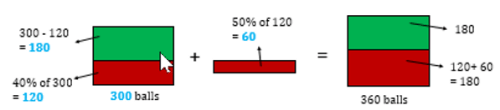The concentration of the ball mix is changed twice in this problem statement. Let us first talk only about the first change-when 50% more red balls are added to the box

The statement ‘50% more red balls’ means ‘50% more than the original number of red balls.’

The original number of red balls is 120

So, number of red balls added = 50% of 120 = 50/100 * 120 = 60

Therefore, Total number of balls in the box now = 300 + 60 = 360

Of these, number of red balls = 120 + 60 = 180

And, number of green balls is still 180

Percent of green balls in the box now = 180/360 * 100 = 50%

So, we still need to increase the number of red balls in the box to decrease the percent of green balls further to 40%

Let the number of red balls added to decrease the number of green balls to 40%, be x.

So, total number of balls in the box now = 360 + x

Number of green balls is still 180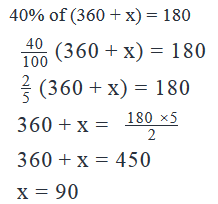Thus, 90 more red balls need to be added.

Step 3: Calculating the final answer

Let 90 be p% of 180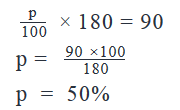Thus, even after the first round, the number of red balls still needs to be increased by a further 50% for the number of green balls to come down to 40%.

Looking at the answer choices, we see that option D is correct

QUESTION: 13

In what ratio did a grocer mix three varieties of tea that cost \$6 per kg, \$7 per kg and \$8 per kg respectively, to make a profit of 10% by selling the mixture at \$7.70 per kg?

(1)  The grocer mixed 135 kg of the variety of tea that costs \$7 per kg.

(2)  The weight of the mixture was 315 kg.

Solution:

Steps 1 & 2: Understand Question and Draw Inferences

We are given that a grocer mixes three varieties of tea in certain amounts to form a mixture. When he sells this mixture at \$7.70/kg, he makes a profit of 10%.

The three varieties of tea cost \$6 per kg (say Type P), \$7 per kg (say Type Q), and \$8 per kg (say Type R).

Let’s say the grocer mixes p kg of Type P, q kg of Type Q and r kg of Type R.

So, the total weight of the mixture = p + q + r

And, the ratio of the three varieties in the mixture = p : q : r

We need to find this ratio. And so, we need to find the values of p, q and r.

Now, the total cost price of the mixture = 6p + 7q + 8r

Thus, the cost price of (p + q + r) kg of the mixture = 6p + 7q + 8r

The cost price of 1 kg of the mixture =C =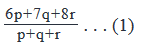We are given that the selling price of 1 kg of the mixture = S = \$7.7

We are also told that Profit = 10%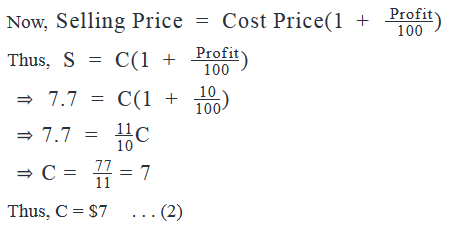Comparing Equations (1) and (2):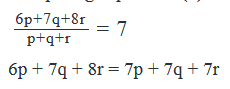Upon simplifying this equation, we get:

p = r       . . . (3)

Thus, we get to know that the Type P and Type R varieties of tea are mixed in equal quantities.

In order to find the ratio p : q : r, we need to know the value of p and q.

Step 3: Analyze Statement 1

The grocer mixed 135 kg of the variety of tea that costs \$7 per kg       Thus,

q = 135     . . . (4)

This statement does not provide any information about the quantity of the P and R types of the teas. So, we can’t find the ratio of different varieties of the teas.

Hence, statement 1 alone is not sufficient to answer the question.

Step 4: Analyze Statement 2

The weight of the mixture was 315 kg

Thus,

p + q + r = 315

2p + q = 315         . . . (5)

Again, the value given is an absolute value for all the three varieties of tea together, but we want the individual values of p and q. Hence, we can’t find the ratio of different varieties of the teas.

Hence, statement 2 alone is not sufficient to answer the question.

Step 5: Analyze Both Statements Together (if needed)

From Statement 1:

q = 135     . . . (4)

From Statement 2:

2p + q = 315         . . . (5)

Now, we have two equations with two unknowns. So, we can easily find the values of p and q.

Thus, the two statements together are sufficient to answer the question.

(Note: If you solve for the value of p and q, you would get: p = 90 kg, q = 135 kg. So, the ratio p : q : r = p : q : p = 90 : 135 : 90 = 2 : 3 : 2)

QUESTION: 14

A milk vendor has 2 cans of milk. The first contains 25% water and the rest milk. The second contains 50% water. How much milk should he mix from each of the containers so as to get 12 litres of milk such that the ratio of water to milk is 3 : 5?

Solution:

Let x and (12-x) litres of milk be mixed from the first and second container respectively
Amount of milk in x litres of the the first container = .75x
Amount of water in x litres of the the first container = .25x
Amount of milk in (12-x) litres of the the second container = .5(12-x)
Amount of water in (12-x) litres of the the second container = .5(12-x)
Ratio of water to milk = [.25x + .5(12-x)] : [.75x + .5(12-x)] = 3 : 5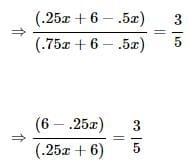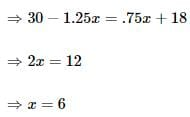Since x = 6, 12-x = 12-6 = 6

Hence 6 and 6 litres of milk should mixed from the first and second container respectively

QUESTION: 15

8 litres are drawn from a cask full of wine and is then filled with water. This operation is performed three more times. The ratio of the quantity of wine now left in cask to that of the water is 16 : 65. How much wine did the cask originally hold?

Solution:

Let initial quantity of wine = x litre

After a total of 4 operations, quantity of wine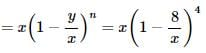Given that after a total of 4 operations, the ratio of the quantity of wine left in cask  to that of water = 16 : 65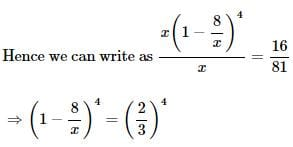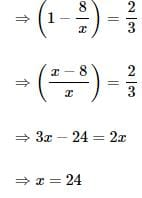Use Code STAYHOME200 and get INR 200 additional OFF Use Coupon Code